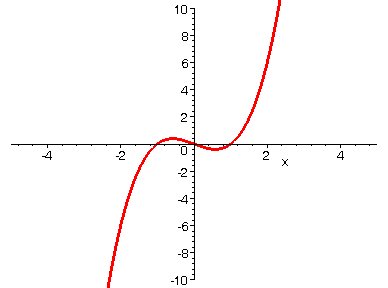VERTICAL STRETCH

If you take a function y = f(x) and replace it by y = k*f(x) where k > 1, then the net result graphically is that the graph of f(x) is stretched vertically away from the horizontal axis. Below we start with a polynomial function of the form y = k*f(x), and the animation shows the effect on the graph as we let k increase from 1 to 20.

> with(plots):

> f:=x->x^3-1*x;> A := seq(plot(i*f(x), x=-5..5, numpoints=500, thickness=3), i=1..20):

> display(A, insequence=true, view=[-5..5, -10..10]);>